# Angle Properties

## Angles at a point

Below are some key angle facts that you need to know off by heart to work out angle problems.

Labelling Angle: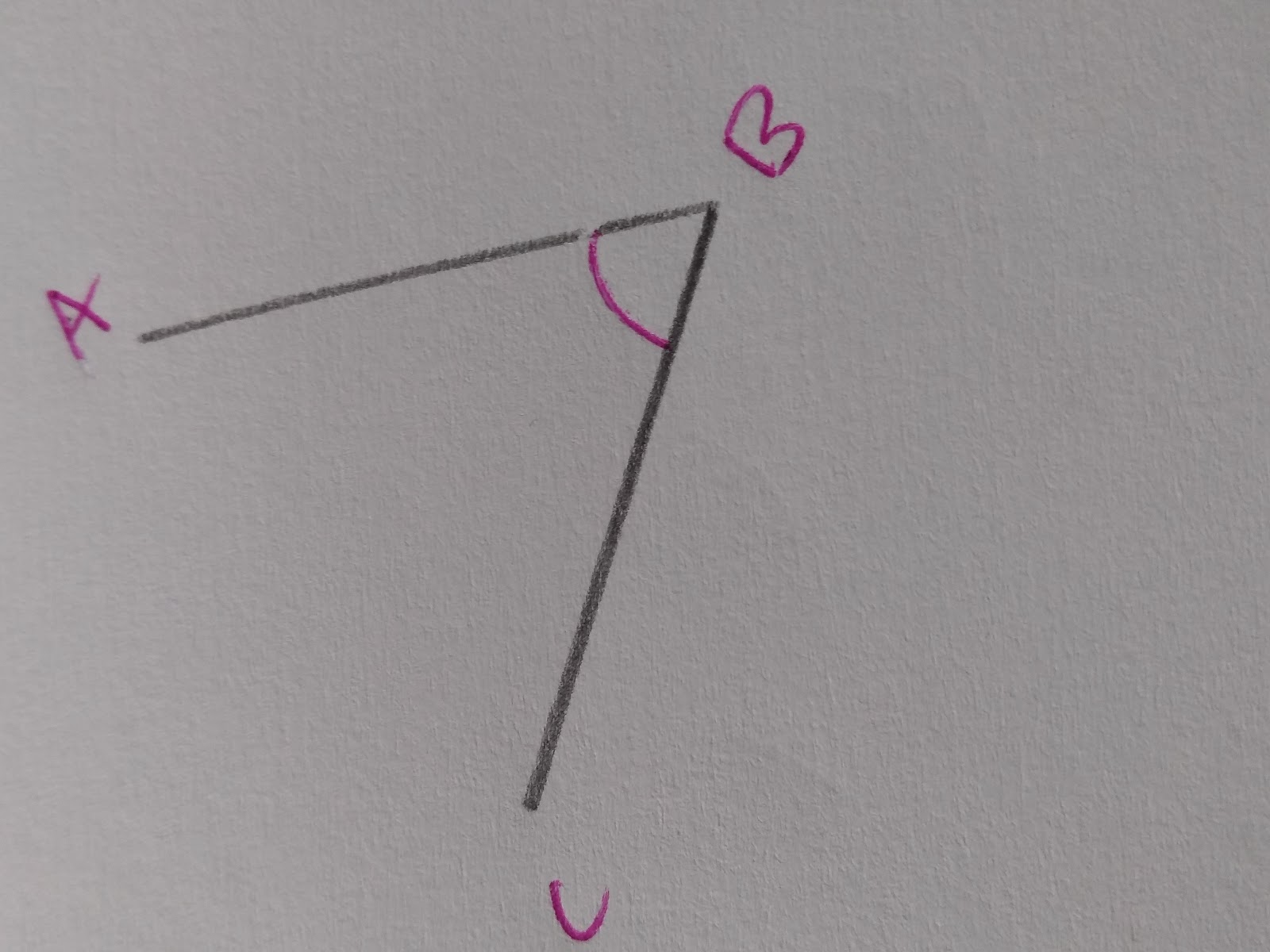This angle is known as ABC. It is formed by two line segments, AB and BC.

Angles at a Point

Angles at a point will always sum to 360 degrees.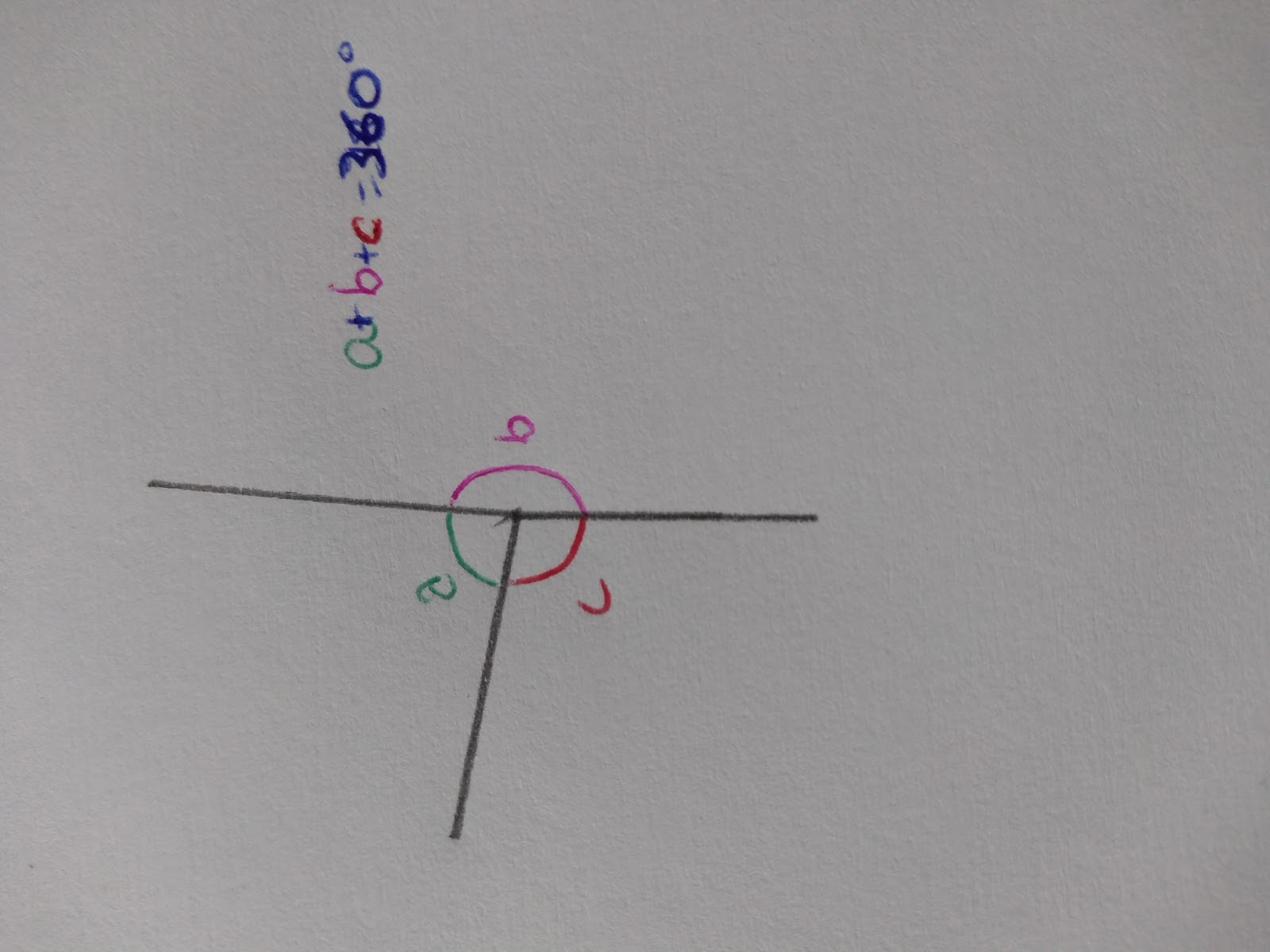## Angles on a straight line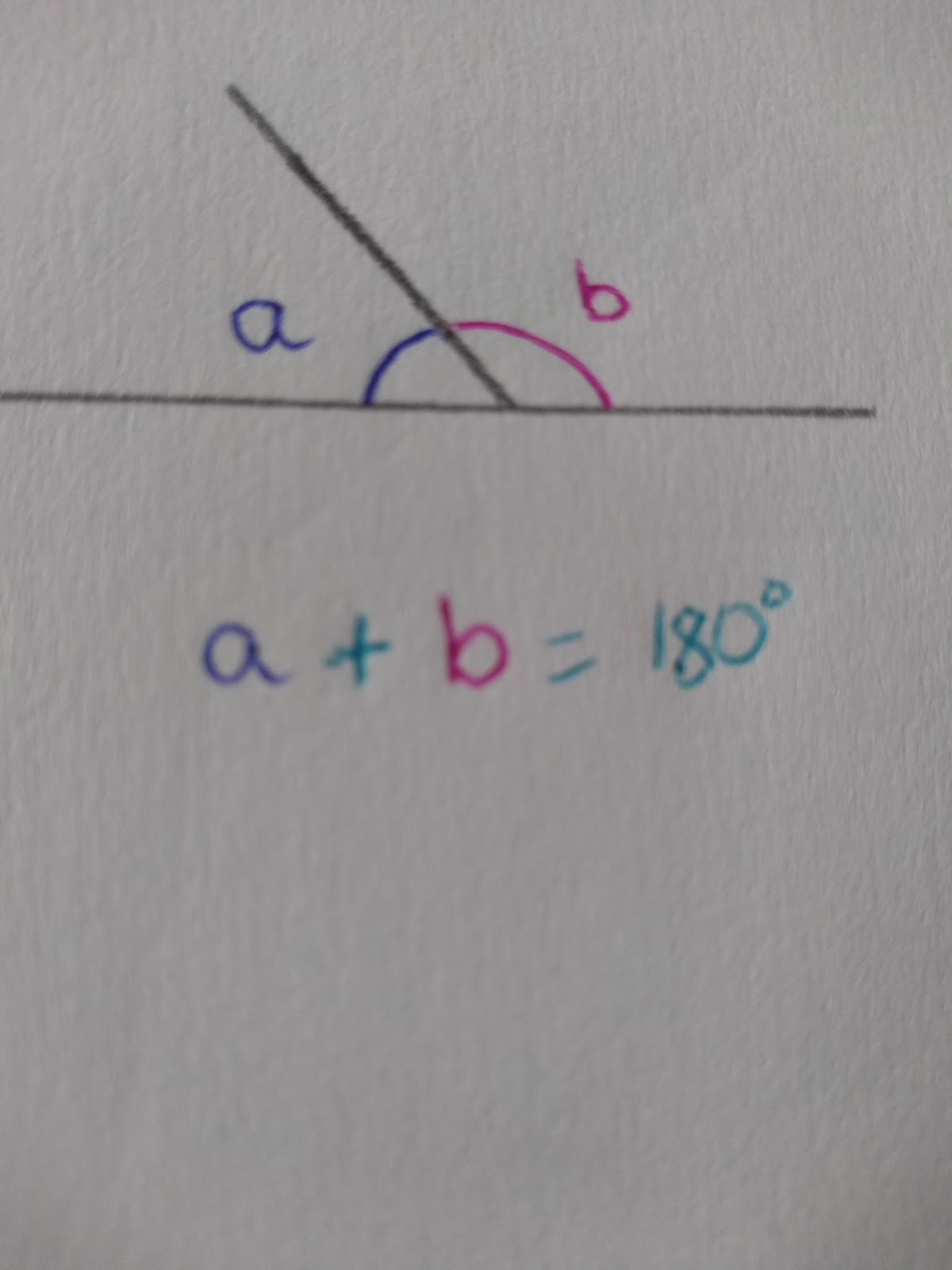Angles on a straight line add to 180 degrees.

## Vertically oppositive angles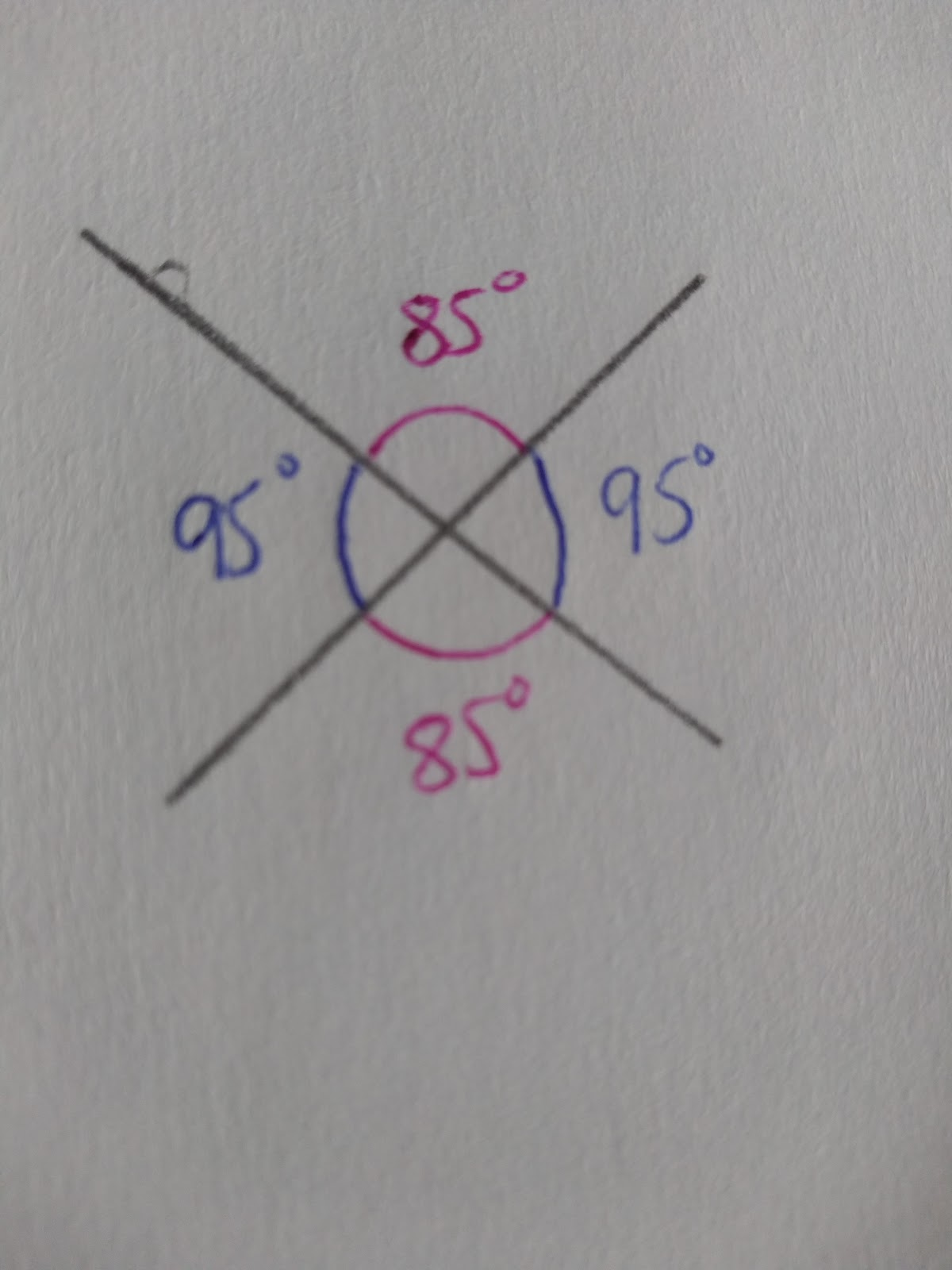## Alternate Angles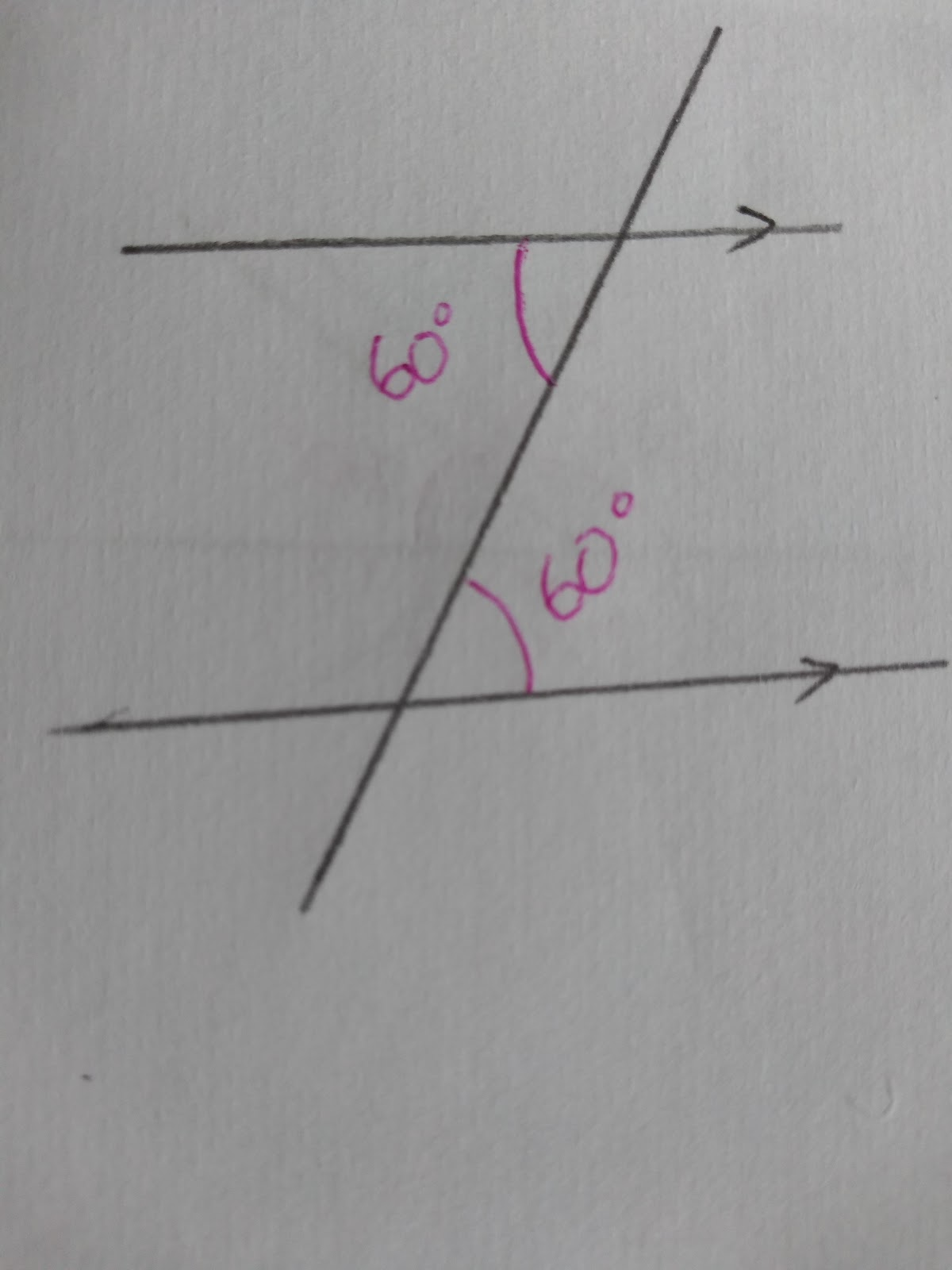## Corresponding Angles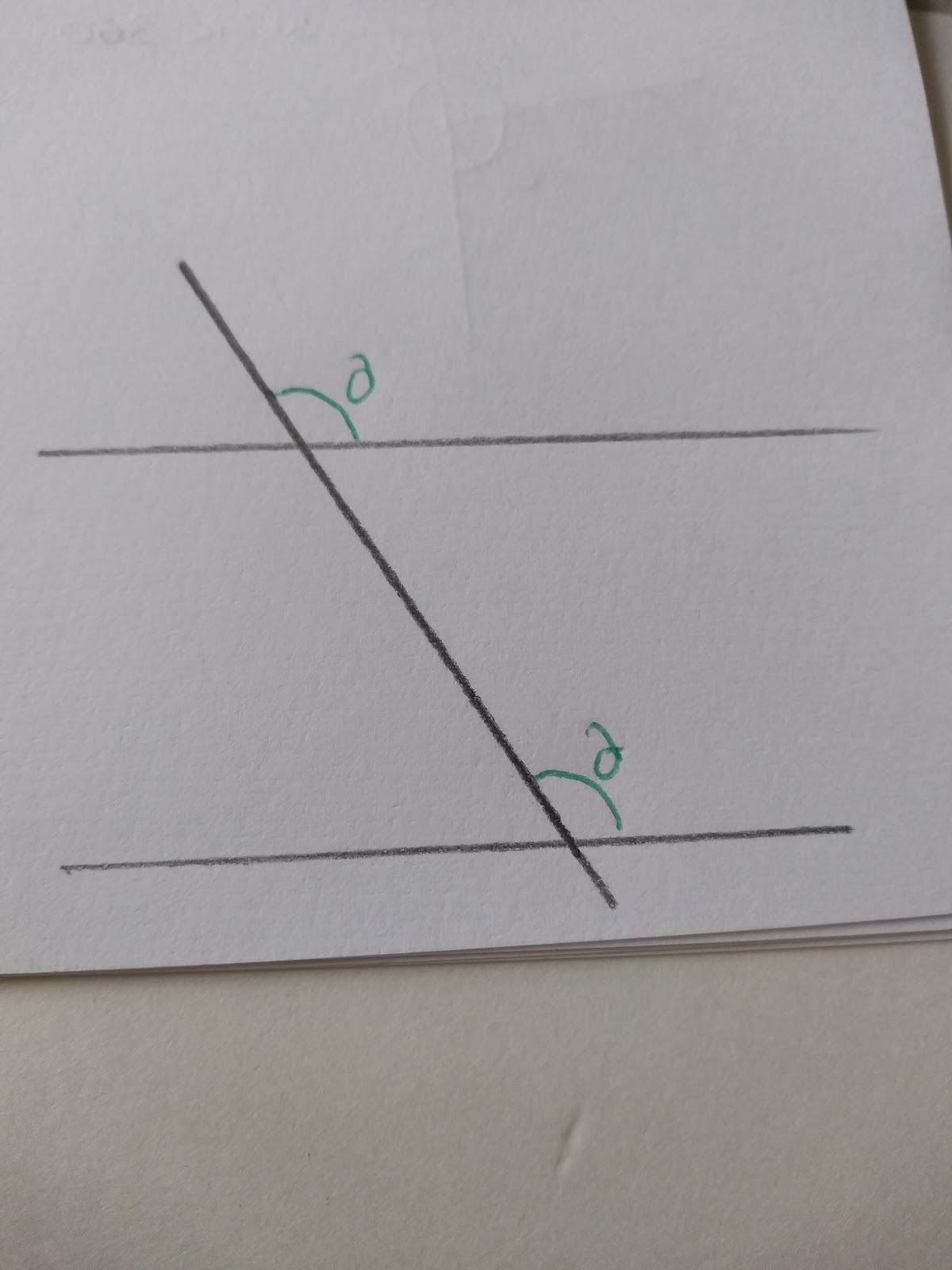## Angles on parallel lines

A key to solving problems with angles on parallel lines is remembering that:

1. Corresponding angles are equal.
2. Alternate angles are equal.
3. Angles in parallel lines always add to 180
4. That angles on a straight line always add to 180

To solve parallel line angle problems.

1. Write down any angles that you know
2. Think through the different types of angles you can find on parallel lines ( corresponding/ alternate)
3. Look to see if there are any other angle facts

## Angles in triangles

Angles in triangles will always add to 180 degrees

Scalene triangles don’t have any equal angles.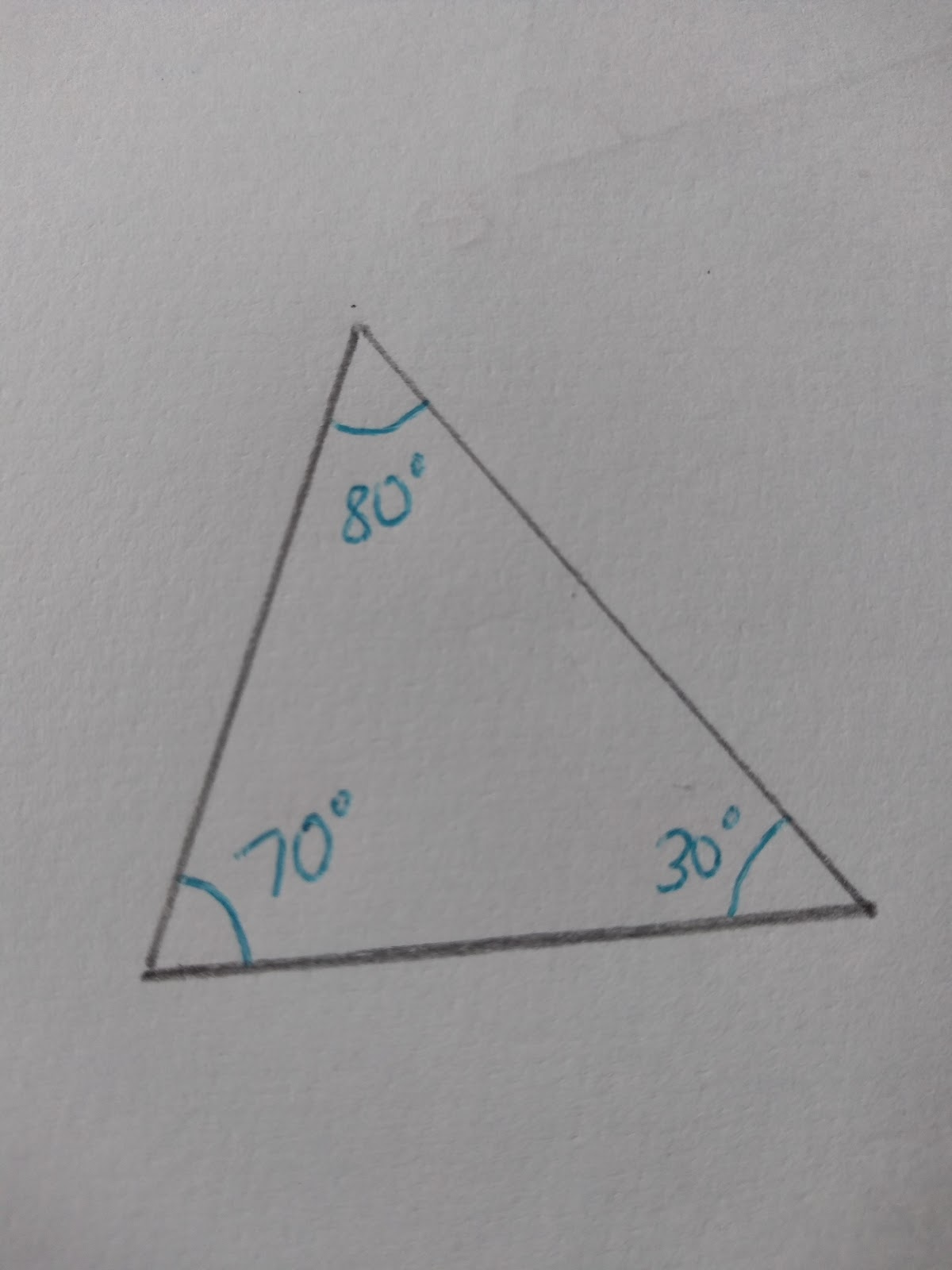All the angles and sides are different!

Isosceles triangles have two equal angles.

Remember to look carefully at the markings, angles and lines are not equal unless you can prove them to be or they have markings that identify that they are!

Equilateral triangles angles are 60 degrees.## Angles in polygons

To find angles inside polygons use the formula

The sum of interior angles = (number of sides-2) x 180

To find each individual angle of a regular polygon you will need to divide by the number of sides.

Eg. How many degrees in a square?

= (4-2) x 180

= 2 x180

= 360

The reason why this works is because we can split the angles in a square into two triangle! This is the case with every polygon.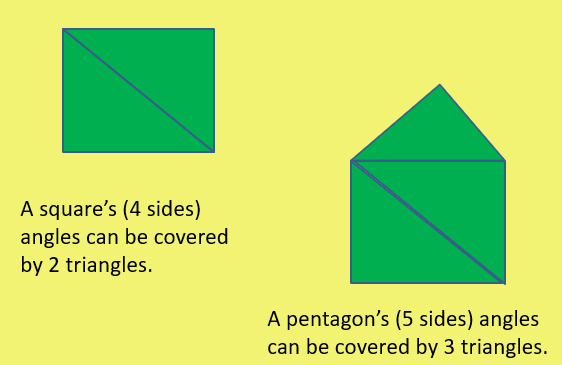This is the case for all regular and irregular polygons.

## Exterior angles in polygons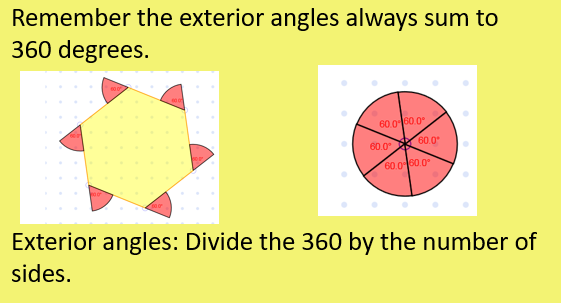The exterior angles will only be equal if the shape is regular.

How many degrees are there around a point?
360
What sort of triangle has two equal angles?
Isosceles
How many degrees are inside a regular hexagon?
720
How many degrees is each exterior angle on a regular pentagon.
72